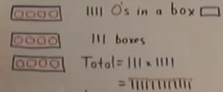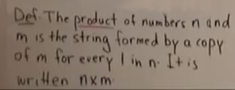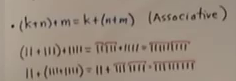## 16 Jun 2019

### Wildberger (2) Math Foundations, 2: “Arithmetic with Numbers”, summary

[Search Blog Here. Index tabs are found at the bottom of the left column.]

[Central Entry Directory]

[Mathematics, Calculus, Geometry, Entry Directory]

[Norman Wildberger, entry directory]

[Wildberger, Math Foundations, entry directory]

[The following is summary of Wildberger’s video lecture. You will find that he is a supremely talented teacher. Any mistakes are my own, as I am not a mathematician.  Bracketed comments are my own and are not to be trusted. If I have any general commentary, it comes at the end.]

Norman J. Wildberger

Course Series

Math Foundations

Math Foundations A (1-79):

Arithmetic and Geometry

2

“Arithmetic with Numbers”(Image source. Wildberger, Math Foundations 2)

Brief summary:

(2.1) Last week we conceived the natural numbers as discrete unities that increase by accretion (adding strokes to sets of strokes. See section 1.5). (2.2) We imagine a boy a hundred thousand years ago sent to a water hole, tasked with counting the animals there. He sees buffalo (represented as squares) and antelope (represented as triangles). The boy then uses the counting procedure from section 1.7 to count the totals of each animal. He does so by making scratches in a piece of bark, thereby fashioning our numerical series of strokes. He counts the buffalo and makes one set of scratches for them, and he counts the antelope and makes another set of scratches for them. The boy returns to his village and shows the people his count. But then they ask him to go back for a total count of all animals. Yet, the boy uses a technique to get the full count without going back to the water hole and repeating the whole counting procedure. The boy first copies the first set of strokes (for the buffalo), and he likewise copies the second set of strokes (for the antelope) right next to the first copied set of strokes. This is adding them. It indicates the total number of animals.(Image source. Wildberger, Math Foundations 2)

This is the operation of summation, specifically the sum of two numbers. It is defined as: the sum of numbers n and m is the combination (the putting together, concatenation) of the strings of ‘|’. We write this as n + m.(Image source. Wildberger, Math Foundations 2)

(2.4) Multiplication is more complicated. We consider a motivating example. We have three boxes, each with four circles. To total them, we combine four plus four plus four.(Image source. Wildberger, Math Foundations 2)

We think of it as ‘||| × ||||’, that is, as three groups of four. So we will replace each of the ones in the first string ‘|||’ with a copy of the second number ‘||||’. That gives us the longer string of twelve strokes: ‘||||||||||||’ (see image above). We thus define multiplication in the following way: The product of numbers n and m is the string formed by a copy of m for every 1 in n. It is written: n × m.(Image source. Wildberger, Math Foundations 2)

(2.5) There are three laws for multiplication. {1} The Commutative Law: n × m = m × m. {2} The Associative Law: (k × n) × m = k × (n × m). {3} The Identity Law (on the board but not spoken): n × 1 = n.(Image source. Wildberger, Math Foundations 2)

{4} The Distributive Laws. These connect addition and multiplication. {4a} k × (n + m) = (k × n) + (k × m). And we introduce an important notational convention here. We say that multiplication takes precedence over addition, and so we eliminate the parentheses: k × (n + m) = k × n + k × m. {4b} (k + n) × m = k × m + n × m.(Image source. Wildberger, Math Foundations 2)

The laws in these two section (including the laws of addition, section 2.3) are the most important ones in all of mathematics. “In fact, they are far more important than almost anything else. They are important because they are simple and because their effect in everyday life is so profound. All of mathematics uses these laws, directly or indirectly, all the time.” We need to understand why these laws are true in an intuitive and non-jargonistic way, in everyday language, using only the notation and conventions that we have so far established. Making such proofs, or reasons, for these laws, is our homework for the next session.

Contents

Online Description

2.1

[Review of Last Week]

2.2

[The Sum of Two Numbers]

2.3

2.4

[Multiplication]

2.5

[Laws for Multiplication]

General Commentary

Bibliography

Online Description [Quoting]:

We introduce the two basic operations on natural numbers: addition and multiplication. Then we state the main laws that they satisfy. This is a basic and fundamental fact about natural numbers; that we can combine them in these two different ways. A lot of arithmetic, and later algebra, comes down to the interaction between addition and multiplication!

Summary

(Repeats the “brief summary” and “contents” above, but with video links)

2.1

[Review of Last Week]

[Last week we conceived the natural numbers as discrete unities that increase by accretion (adding strokes to sets of strokes. See section 1.5).(Image source. Wildberger, Math Foundations 2)]

(0.08-next)

[ditto]

[contents]

2.2

[The Sum of Two Numbers]

[We imagine a boy a hundred thousand years ago sent to a water hole, tasked with counting the animals there. He sees buffalo (represented as squares) and antelope (represented as triangles). The boy then uses the counting procedure from section 1.7 to count the totals of each animal. He does so by making scratches in a piece of bark, thereby fashioning our numerical series of strokes. He counts the buffalo and makes one set of scratches for them, and he counts the antelope and makes another set of scratches for them. The boy returns to his village and shows the people his count. But then they ask him to go back for a total count of all animals. Yet, the boy uses a technique to get the full count without going back to the water hole and repeating the whole counting procedure. The boy first copies the first set of strokes (for the buffalo), and he likewise copies the second set of strokes (for the antelope) right next to the first copied set of strokes. This is adding them. It indicates the total number of animals.(Image source. Wildberger, Math Foundations 2)

This is the operation of summation, specifically the sum of two numbers. It is defined as: the sum of numbers n and m is the combination (the putting together, concatenation) of the strings of ‘|’. We write this as n + m.(Image source. Wildberger, Math Foundations 2)]

(1.03-next)

[ditto]

[contents]

2.3

[There are certain facts that we can discover about the addition operation, and these are the laws of addition. {1} We first see that it is commutative: n + m = m + n. We can see this in an illustration where we have five strokes and two strokes, which total seven strokes, and otherwise two strokes and five strokes, which also, in a readily visible way, totals the same amount of seven strokes.(Image source. Wildberger, Math Foundations 2)

{2} The second one is the associative law: (k + n) + m = k + (n + m). As we see, this one involves three values, k, m, and n. This gives us two options for making couples of addition. We can see, again, how this works with the strokes, because we neither increase no decrease the total number of stroke-objects, and so grouping them differently has no effect on the total sum.(Image source. Wildberger, Math Foundations 2)

{3} The third law is a minor one. We can write the successor operation s(n) as n + 1.(Image source. Wildberger, Math Foundations 2)]

(3.50-next)

[ditto]

[contents]

2.4

[Multiplication]

[Multiplication is more complicated. We consider a motivating example. We have three boxes, each with four circles. To total them, we combine four plus four plus four.(Image source. Wildberger, Math Foundations 2)

We think of it as ‘||| × ||||’, that is, as three groups of four. So we will replace each of the ones in the first string ‘|||’ with a copy of the second number ‘||||’. That gives us the longer string of twelve strokes: ‘||||||||||||’ (see image above). We thus define multiplication in the following way: The product of numbers n and m is the string formed by a copy of m for every 1 in n. It is written: n × m.(Image source. Wildberger, Math Foundations 2)]

(06.23-next)

[ditto]

[contents]

2.5

[Laws for Multiplication]

[There are three laws for multiplication. {1} The Commutative Law: n × m = m × m. {2} The Associative Law: (k × n) × m = k × (n × m). {3} The Identity Law (on the board but not spoken): n × 1 = n.(Image source. Wildberger, Math Foundations 2)

{4} The Distributive Laws. These connect addition and multiplication. {4a} k × (n + m) = (k × n) + (k × m). And we introduce an important notational convention here. We say that multiplication takes precedence over addition, and so we eliminate the parentheses: k × (n + m) = k × n + k × m. {4b} (k + n) × m = k × m + n × m.(Image source. Wildberger, Math Foundations 2)

The laws in these two section (including the laws of addition, section 2.3) are the most important ones in all of mathematics. “In fact, they are far more important than almost anything else. They are important because they are simple and because their effect in everyday life is so profound. All of mathematics uses these laws, directly or indirectly, all the time.” We need to understand why these laws are true in an intuitive and non-jargonistic way, in everyday language, using only the notation and conventions that we have so far established. Making such proofs, or reasons, for these laws, is our homework for the next session.]

(07.35-end)

[ditto]

[contents]

In the case of the laws of addition (2.3), we can see the value of Wildberger’s stroke-representation approach. For instance, for the commutative law (n + m = m + n), we can visually see the identity between the two sets of stroke additions.(Image source. Wildberger, Math Foundations 2)

This law seems in a sense to be a property of the line objects themselves, which could be easily discovered by playing with them in a spontaneous way. For instance, if we had a group of stroke-like objects, and we divided them into two groups, we would find that no matter how we made those divisions, we still in total will have the same number of objects, as none will have disappeared or come into existence out of nowhere. And more to the point, we will see that simply by moving the divided groups around changes nothing in the total number. This sort of obvious mode of demonstration would seem to work for the multiplication laws as well, and thus Wildberger is fulfilling his characterization of mathematics as something natural, intuitable, coherent, and tied to reality (see section 1.4 and the General Commentary to that first lesson.)

I would like to continue with some assumptions we discussed last time in the general commentary. We wanted to know the grounds of equality. And we said that in our intuition and observations of the world, there seems to be no such thing at least in an objective sense. However, we said that in certain situations, two things that are not equal can be unequal to a degree that in a particular context is negligible, and so for all practical purposes they are equal for us. Two objects on a balancing scale may show on the read-out to be the exact same weight, but on a more sensitive scale they would show to be a little off. But insofar as the first scale is sensitive enough for our needs, the two are minimally unequal enough to be treated as equal. I mention that, because we missed an important assumption in the last commentary that comes more to light with the buffalo/antelope example from section 2.2. Suppose the story went the other way. The boy’s task is to count the total animals first, which he does.He thus first ignores the differences between buffalo and antelope. But next, he is asked for a specific count of each animal type. Now he makes that distinction, giving strokes in different groupings for the different types of animals. Here, while the groupings are thought to be distinct, the items in each group are all homogeneously repetitions of strokes. Suppose further that the boy’s third task is to be more precise, and to give counts of all the male antelopes versus female, and all the male buffalo versus female. Now he will have four groups of strokes, noticing more differences. And this can be repeated. What we might observe here is the denuding of real things of their particularities and representing them with strokes. This is an assumption we should now consider, namely, that any particular object can be represented by a quantifying symbol that is homogeneous with the quantifying symbols given to any other object. The basis for this, it seems to me, is the widening of generalities of types. We can think of animals, more generally, living things, then physical things, and most generally of entities of any sort whatsoever, either existant or not. The question is whether or not this is a falsification of reality; for, there are no things either in the imagination or in the real world that have no particularizing properties of any sort. What is a thing with no properties whatsoever? There is no such thing. But what are the strokes representing the animals? They are things with no particularizing properties of any sort. Nonetheless, we know that the natural numbers exist in nature (see this entry on how they are exhibited in waves). At this moment, I am not sure where to go with this, but I will try to come back to it. Perhaps natural numbers in the natural state should be understood as, to give some possibilities: abstractions like our strokes, but in a cosmic consciousness that pervades all things; ratios between differences of physical quantity, somehow; structural relationships of some sort that can be understood in terms of numerical quantities but in themselves are simply natural structuring relationships of some sort; or, the physical world is like a giant computer, and somehow fundamentally all things are number or ratio, but on the phenomenal level they appear as non-numerical.]

Wildberger, Norman J. (2). “Arithmetic with Numbers”.  Part 2 of the course series:  Math Foundations. Video.

https://youtu.be/-96tlu_sShM

Math Foundations A (1-79)

Math Foundations B (80-149)

Math Foundations C (150 - )

[njwildberger]

Insights into Mathematics